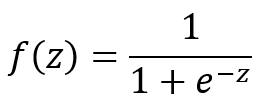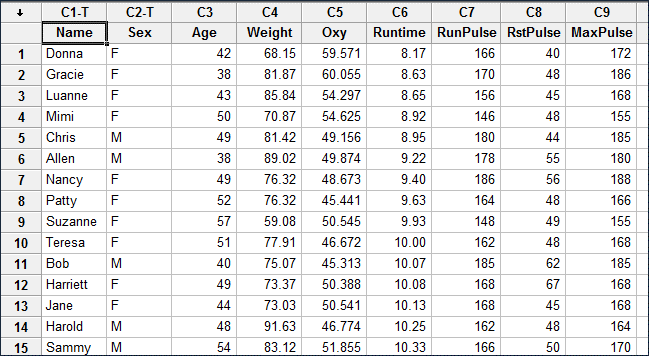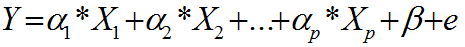# Tag Archives | Regression## Logistic Regression with JMP

What is Logistic Regression? Logistic regression is a statistical method to predict the probability of an event occurring by fitting the data to a logistic curve using logistic function. The regression analysis used for predicting the outcome of a categorical dependent variable, based on one or more predictor variables. The logistic function used to model [...]## Logistic Regression with Minitab

What is Logistic Regression? Logistic regression is a statistical method to predict the probability of an event occurring by fitting the data to a logistic curve using logistic function. The regression analysis used for predicting the outcome of a categorical dependent variable, based on one or more predictor variables. The logistic function used to model [...]## Logistic Regression with SigmaXL

What is Logistic Regression? Logistic regression is a statistical method to predict the probability of an event occurring by fitting the data to a logistic curve using logistic function. The regression analysis used for predicting the outcome of a categorical dependent variable, based on one or more predictor variables. The logistic function used to model [...]## Stepwise Regression with JMP

What is Stepwise Regression? Stepwise regression is a statistical method to automatically select regression models with the best sets of predictive variables from a large set of potential variables. There are different statistical methods used in stepwise regression to evaluate the potential variables in the model: F-test T-test R-square AIC Three Approaches to Stepwise Regression [...]## Stepwise Regression with Minitab

What is Stepwise Regression? Stepwise regression is a statistical method to automatically select regression models with the best sets of predictive variables from a large set of potential variables. There are different statistical methods used in stepwise regression to evaluate the potential variables in the model: F-test T-test R-square AIC Three Approaches to Stepwise Regression [...]## Multiple Linear Regression with Minitab

What is Multiple Linear Regression? Multiple linear regression is a statistical technique to model the relationship between one dependent variable and two or more independent variables by fitting the data set into a linear equation. The difference between simple linear regression and multiple linear regression: Simple linear regression only has one predictor. Multiple linear regression [...]## Multiple Linear Regression with SigmaXL

What is Multiple Linear Regression? Multiple linear regression is a statistical technique to model the relationship between one dependent variable and two or more independent variables by fitting the data set into a linear equation. The difference between simple linear regression and multiple linear regression: Simple linear regression only has one predictor. Multiple linear regression [...]## Multiple Linear Regression with JMP

What is Multiple Linear Regression? Multiple linear regression is a statistical technique to model the relationship between one dependent variable and two or more independent variables by fitting the data set into a linear equation. The difference between simple linear regression and multiple linear regression: Simple linear regression only has one predictor Multiple linear regression [...]